##### What Do make_timestamp() and make_timestamptz() Functions do in PostgreSQL?

To show the timestamps we use the make_timestamp() and make_timestamptz() functions. The make_timestamp() function returns the timestamp without the timezone according to the input values and the make_timestamptz() function returns the timestamp with the time zone according to the parameters passed into the function. Both functions give errors when the input parameters have some issue maybe due to the wrong data type or due to out-of-range parameters.

This article will show how the make_timestamp() and make_timestamptz() functions work. Let’s get started.

What Do make_timestamp and make_timestamptz() Functions do in PostgreSQL?

What these functions have in common is that they take parameters as input, make_timestamp() takes 6 parameters while make_timestamptz() takes 7 parameters of which 6 are required and one is optional, and then returns a value with the specific data type. The return type of value in the case of the make_timestamp() function is the simple timestamp without a time zone and in the case of the make_timestamptz() function is a timestamp with a time zone.

Let's discuss the make_timestamp() function first.

The make_timestamp() Function in PostgreSQL

The make_timestamp() function manipulates the parameter it takes and converts it into a timestamp without a time zone. These parameters are 6 in number: Year, Month, Day, Hour, Minute, and Second. Remember that the sequence of these parameters has to be the exactly same otherwise, this could cause an error. The basic syntax for this function is:

`make_timestamp(Year INT, Month INT, Day INT, Hour INT, Minute INT, Second DOUBLE PRECISION);`

All these parameters are required and the type of all of them should be “Integer” except second. The “second” parameter has to be of DOUBLE PRECISION data type.

Let’s move towards an example to see how this function works

Example

Consider the following query as an example:

`SELECT make_timestamp(2023, 8, 24, 11, 31, 17.43);`

The function in the above query will convert the parameters into a proper timestamp format as follows: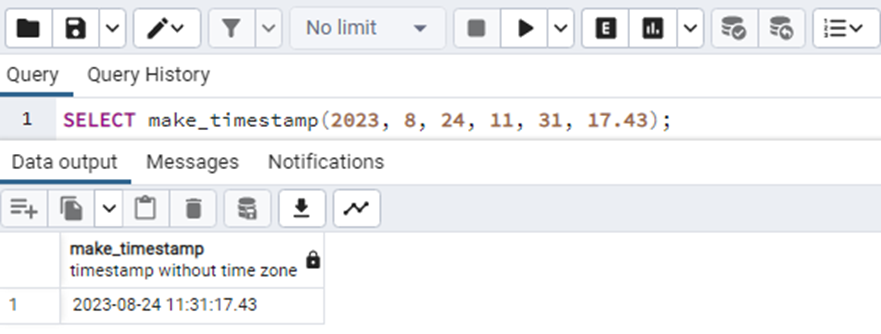In the above output, you can see that the returned type of the function is a timestamp without a time zone. We can verify it by using the “pg_typeof()” function. The “pg_typeof()” function takes an argument and returns us the data type of that argument.

The query will look like this:

`SELECT pg_typeof(make_timestamp(2023, 8, 24, 11, 31, 17.43));`

The output of the above query will clearly show the returned type: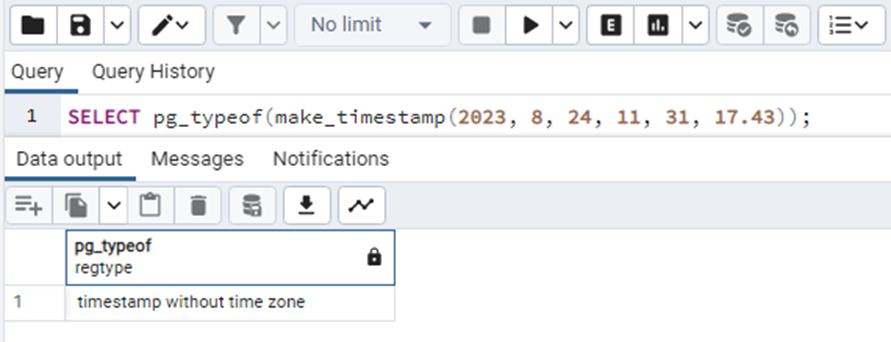So the make_timestamp() function always returns the value having data type “timestamp without time zone”.

Consider another example in which we will intentionally replace the value of a month parameter greater than 12 let's say it is 21. Let's see how will the query respond to this case.

`SELECT make_timestamp(2023, 21, 24, 11, 31, 17.43);`

When we execute this query, the output will be: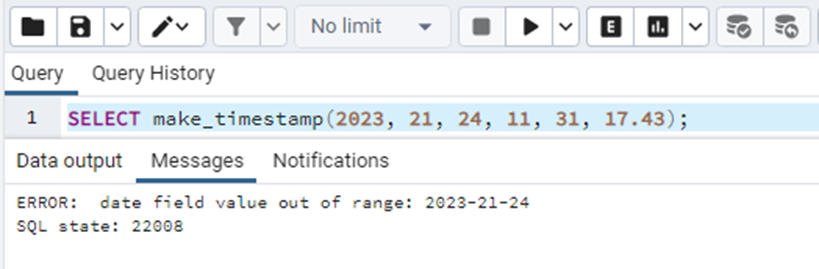The query gives an “out of range” error. This is because the value of the parameter month is more than 12 as the month in a year can not be greater than 12. The same case will happen to the other values.

Special case

As we have discussed above, the data type of each parameter should be INT and for the second it should be DOUBLE PRECISION. According to PostgreSQL documentation, it should be INT and DOUBLE PRECISION but there is a special case. If we pass the arguments in strings this also seems to work without any issue as soon as the values are in their proper ranges. This may be because they can be implicitly converted into integers. Let’s see an example of this special case.

`SELECT make_timestamp('2023', '8', '24', '11', '31', '17.43');`

By executing the above query we will get: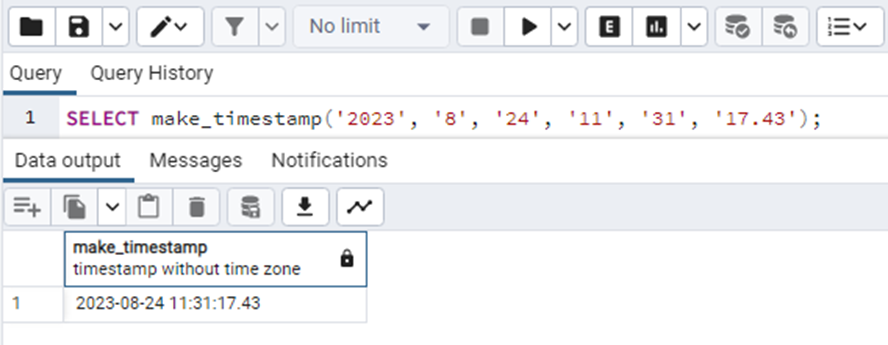This means that under this condition the query also works properly.

But you have to make sure about one thing, the arguments must be valid to be converted into the INT. For example, if we execute the following query:

`SELECT make_timestamp('2023', 'August', '24', '11', '31', '17.43');`

It will give an error that: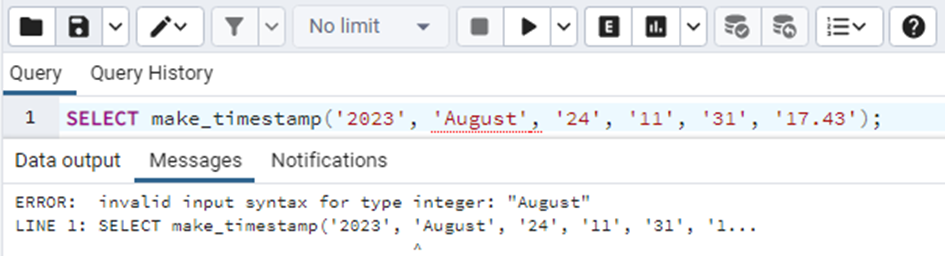This is because the “August” is not valid and can not be converted into an integer lately.

That was all about make_timestamp() function. Now we will move towards the make_timestamptz() function.

The make_timestamptz() Function in PostgreSQL

The make_timestamptz() function also works almost the same as make_timestamp(). It manipulates the parameter it takes into the timestamp with the time zone. These parameters are 7 in number: year, month, day, hour, min, sec, and timezone text. Remember that the sequence of these parameters has to be the exactly same otherwise, this could cause an error. The basic syntax for this function is:

`make_timestamp(Year INT, Month INT, Day INT, Hour INT, Minute INT, Second DOUBLE PRECISION, [ timezone text ]);`

Note that, the first 6 parameters are required but the last parameter i.e. timezone text is optional. If this text is not passed, the current time zone is used.

All the parameter types should be “Integer” while for a second it has to be a “DOUBLE PRECISION”. The return value of the query is a timestamp with time zone.

Now let's move towards an example.

Example

Consider the following query as an example:

`SELECT make_timestamptz(2023, 8, 24, 11, 44, 16.46,'Indian/Mauritius');`

The function in the above query will convert the parameter into a proper timestamp with Indian timezone format as follows: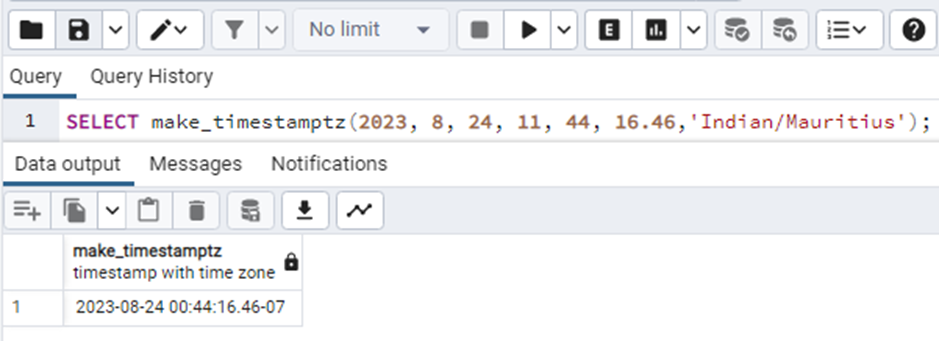In the above output, you can see that the returned type of the function is time with time zone. We can verify it by using the “pg_typeof()” function.

`SELECT pg_typeof(make_timestamptz(2023, 8, 24, 11, 44, 16.46));`

The output of the above query will clearly show the returned type: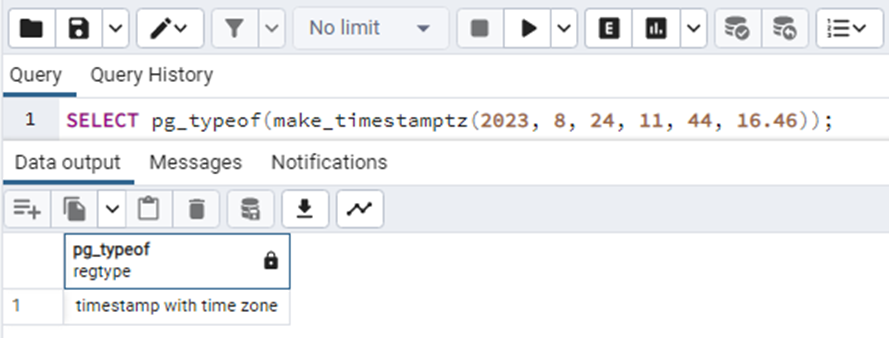Consider another example in which we will intentionally replace the value of a sec parameter greater than 60 let's say it is 78.45. Let's see how will the query respond to this case.

`SELECT make_timestamptz(2023, 8, 24, 11, 44, 78.45,'Indian/Mauritius');`

When we execute this query, the output will be: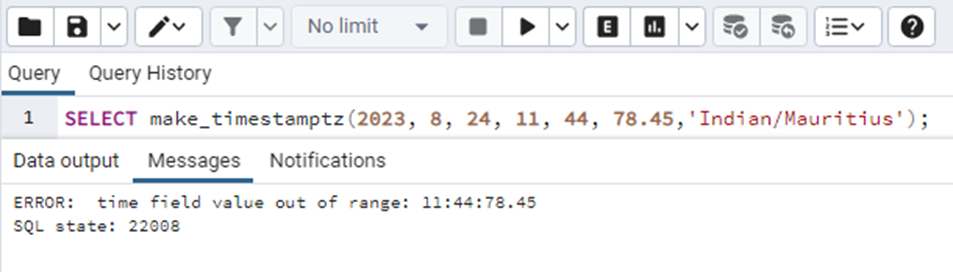The query gives an “out of range” error. This is because the value of parameter sec is more than 60 as the seconds in a minute can not be greater than 60. The same case will happen to the other values as well.

Special case

Similar to the make_timestamp() function, this function also has a special case. As we have discussed above, the data type of all parameters should be INT and for the second it is DOUBLE PRECISION. According to PostgreSQL documentation, they should be INT and DOUBLE PRECISION but there is a special case. If we pass the arguments in strings this also seems to work without any issue as soon as the values are in their proper range. Let’s see an example of this special case.

`SELECT make_timestamptz('2023', '8', '24', '11', '50', '18.45');`

By executing the above query we will get: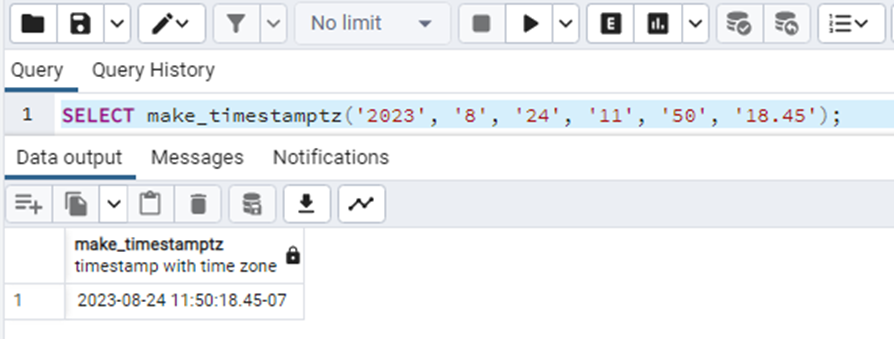This means that under this condition the query also works properly. But if any of the arguments exceeds its range, it will give the out-of-range error. Here you can also notice one thing I haven’t specified any timezone, so it has taken the indian timezone because currently it is the indian timezone.

Conclusion

There are many functions in PostgreSQL used to manipulate the time. The functions make_timestamp() and make_timestamptz() are significant among those and are used to get the timestamps without and with the time zones respectively.